# ISERROR Excel FunctionArticle byExcelMojo Team## What Is ISERROR Function In Excel?

The ISERROR function in Excel is an inbuilt Information function that returns TRUE if the specified value is an error and FALSE otherwise. It works for errors such as #DIV/0!, #NAME?, #NULL, #NUM!, #N/A, #REF!, #SPILL!, #CALC!, and #VALUE!.

Users can use the ISERROR Excel function with IF() to check for errors in spreadsheets containing multiple formulas. It enables them to ensure proper data evaluation according to their requirements. For example, the below table contains a set of data in column A.

Suppose we must check whether each cell in the cell range A2:A12 contains an error type and display the outcome in column B. Then, considering the ISERROR Excel function logic, we can apply the ISERROR() in the specific target cells to get the required output.

In the above ISERROR Excel function example, the function return value is TRUE in the target cells B3, B5, B6, B8, B11, and B12. It is because the source data in the corresponding cells in column A contain error values. On the other hand, the ISERROR Excel function returns FALSE in those target cells where the input data is not an error value.

###### Key Takeaways
• The ISERROR Excel function output is TRUE if the given value is an error, such as #DIV/0!, #NAME?, #NULL, #NUM!, #N/A, #REF!, and #VALUE!. Otherwise, it returns FALSE.
• Users can apply the ISERROR() with the IF() to check errors in worksheets containing many formulas and mathematical calculations.
• Typically, the formula accepts one cell address at a time to return a logical value. But we can use the ISERROR() to check for errors in an array range by executing it as an array formula using the keys Ctrl + Shift + Enter.

### ISERROR() Excel Formula

The ISERROR Excel function syntax is:

where,

• value: The value or expression you require to test.

The argument value in the ISERROR Excel formula is mandatory and typically a cell address.

Further, adhering to the below points can help you avoid the situation of the ISERROR Excel function not working.

• The ISERROR() only checks if the given value or expression is an error or returns an error.
• The ISERROR Excel formula return value is a logical value, TRUE or FALSE.
• The argument value can be numeric, text, mathematical formula, or function.

### How to Use ISERROR Excel Function?

The following steps show how we can apply the ISERROR Excel function.

1. First, ensure we know the cell address of the expression or value we require to check for error.
2. Then, select the target cell and enter the ISERROR() with the cell address of the value we need to check for error.
3. Finally, press Enter to view the ISERROR Excel function return value.

Please Note: You can check for error values in an array range using the ISERROR(). But for that, you must select the target cell range and provide the argument value as the specific array range you need to check for errors while entering the function. And when executing the ISERROR(), apply it as an array Excel formula using the keys Ctrl + Shift + Enter.

The following illustration explains the ISERROR Excel function logic and the abovementioned steps to apply it.

Suppose the below table contains two sets of values (columns A and B), and the results of the mathematical operations performed using them in column D.

And we need to check if the values in column D are error values and display the outcome in column F. Then, we can apply the ISERROR() in the specific target cells.

1. Select the target cell F2, enter the following ISERROR(), and press Enter.

=ISERROR(D2)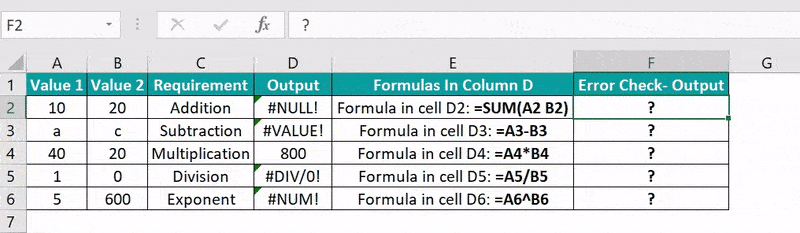Alternatively, we can select the target cell F2 and click FormulasMore FunctionsInformationISERROR to apply the function using the option in the Formulas Tab. This action will open the Function Arguments window.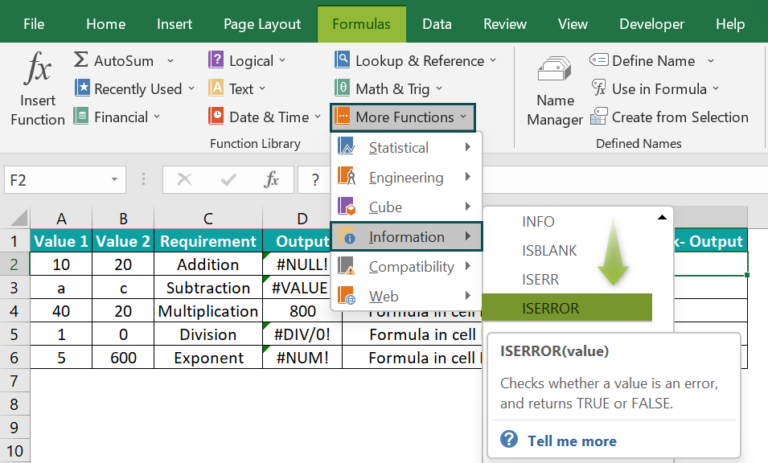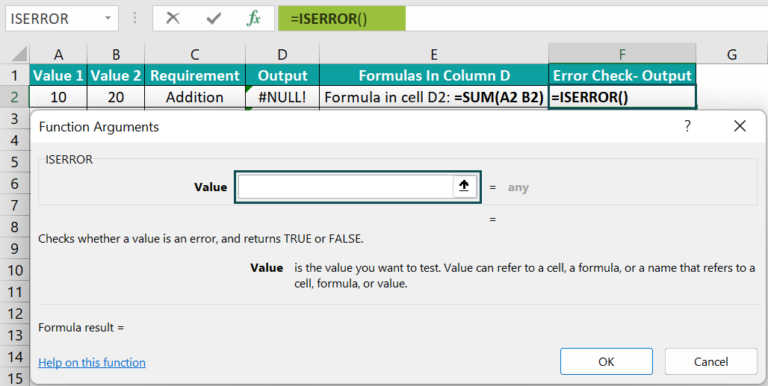Now fill the Value field with the required cell address, D2, in the Function Arguments window.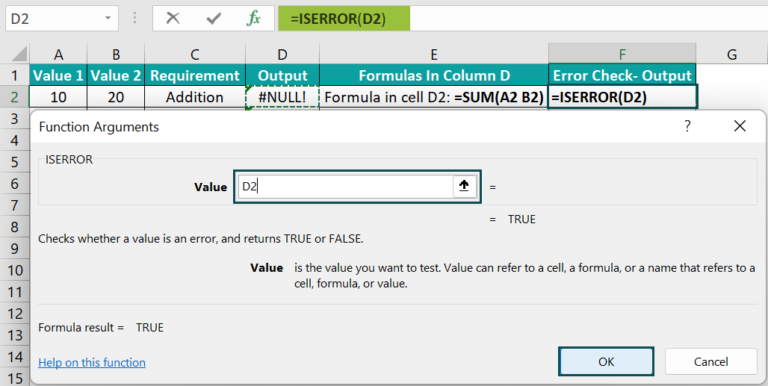And once we click OK in the Function Arguments window, the ISERROR() gets executed in the target cell F2.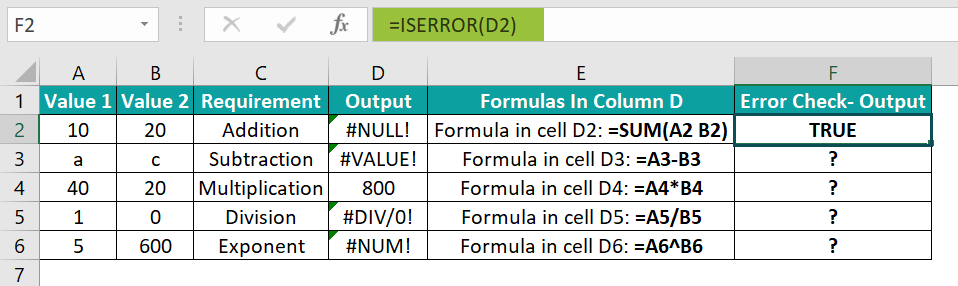2. Drag the fill handle downwards to copy the formula in cells F3:F6.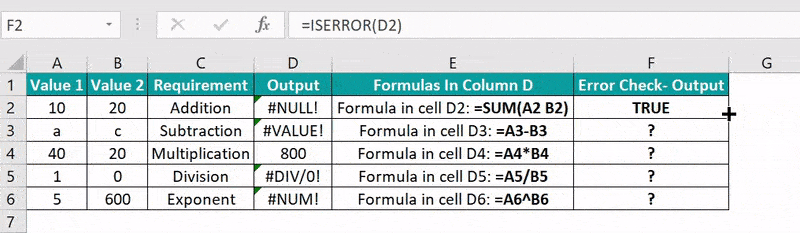In the above ISERROR Excel function example, the input for the ISERROR() in column F cells is the corresponding column D cell address. And the function returns TRUE for the error values in cells D2, D3, D5, and D6. On the other hand, the product of the row 4 values, 40 and 20, is 800 and not an error value. So the ISERROR() output in the target cell D4 is FALSE.

### Examples

The below examples show how you can apply the function properly to avoid the scenario of the ISERROR Excel function not working.

#### Example #1

This example shows how to apply the ISERROR Excel function with IF() to achieve the desired results.

Consider the following table. It contains the cost details of an inventory.

Suppose we must determine the number of units ordered in column C, based on the cost information available in columns A and B, knowing that the source data has issues. Then we can use the ISERROR Excel function with IF() to display the required data in column C and highlight the target cells with data issues.

• Step 1: Select the target cell C2, enter the following IF() containing the ISERROR(), and press Enter.

=IF(ISERROR(A2/B2),”Data Issue”,A2/B2)

• Step 2: Drag the fill handle downwards to copy the formula in cells C3:C10.

In this example, the source data in rows 3, 7, 8, and 10 have data issues. So, when we use these values in the division formula in column C, the formula output is an error value, either due to the numerator or denominator. For instance, when we divide the two given values in rows 3 and 8, the output is the #DIV/0! error. And in the case of rows 7 and 10, the output obtained by dividing the given values is the #VALUE! error.

Now the IF() containing the ISERROR() helps highlight the data issue in the cells mentioned above.

Let us see how the formula works using the target cell C10 expression. First, the term A10/B10 returns the #VALUE! error, as the denominator is not valid data. So, as the input of the ISERROR() is an error value, the function returns TRUE. And as the IF condition is true, the function returns the value Data Issue as the output in the target cell C10.

Thus, when we use the ISERROR() with IF Excel function, you can display customized messages in the cells containing error values instead of showing the errors.

#### Example #2

Here is how the ISERROR Excel function works when applied with the VLOOKUP().

Consider that column A contains a list of quiz participants. And the adjacent table contains a list of students, which includes a few of the quiz participants.

Suppose we need to update the participation status for each student and display the output in column E. Then, we can apply the IF() containing the ISERROR Excel function and VLOOKUP() in the target cells.

• Step 1: Select the target cell E3, enter the below formula, and press Enter.

=IF(ISERROR(VLOOKUP(D3,\$A\$2:\$A\$11,1,FALSE)),”Not Participated”,”Participated”)

• Step 2: Drag the fill handle downwards to copy the formula in cells E4:E17.

Let us see how the formula executes using the target cell E17 formula. First, the VLOOKUP() looks up the name in cell D17, Ruth Robbins, in column A. As the name is not present in the quiz participants list, the VLOOKUP() returns the #N/A error. So, the argument value supplied to the ISERROR() is the #N/A error; hence it returns TRUE. Finally, as the IF condition is true, the IF() returns Not Participated as the output.

#### Example #3

We can utilize the ISERROR Excel function to count the number of errors in a cell range.

The below table contains two sets of inputs in columns A and B and the result of the mathematical operation performed using them in each row in column C.

Suppose the requirement is to determine the total error count in column C and display the result in cell B10. Then we can use the ISERROR within the SUMPRODUCT Excel function in the specific target cell and get the required count.

• Step 1: Select the target cell B10, enter the below formula, and press Enter.

=SUMPRODUCT(–ISERROR(C2:C8))

Here is how the above formula works. As we use the double unary operator, the TRUE and FALSE values in the ISERROR() output, {TRUE;TRUE;FALSE;TRUE;FALSE;FALSE;TRUE}, translate into ones and zeros. The TRUE values in the array range indicate the inputs that are error values in the cell range C2:C8. And then, the SUMPRODUCT() adds the ones and zeros in the above array range, thus returning the value 4.

### Important Things to Note

• The ISERROR Excel function determines if the given expression is an error value or returns an error term.
• The ISERROR() can only return logical values, TRUE or FALSE.
• The ISERROR() argument value can be a numeric value, text, cell reference in Excel to a numeric or text value, function, or mathematical formula.
• We can achieve excellent results using the ISERROR() function with other inbuilt functions, such as VLOOKUP, SUMPRODUCT, and MATCH in Excel.
• The ISERROR excel function accepts one mandatory argument, value, as input.

1. How to use ISERROR() with the MATCH function?

We can use the ISERROR() with the MATCH function in the following way.
Let us see the steps with an example.

The following image shows two tables. The first table contains a list of athletes who qualified for Round 1 in an event. And the second table contains the complete list of athletes who participated in the Round 1 of the event.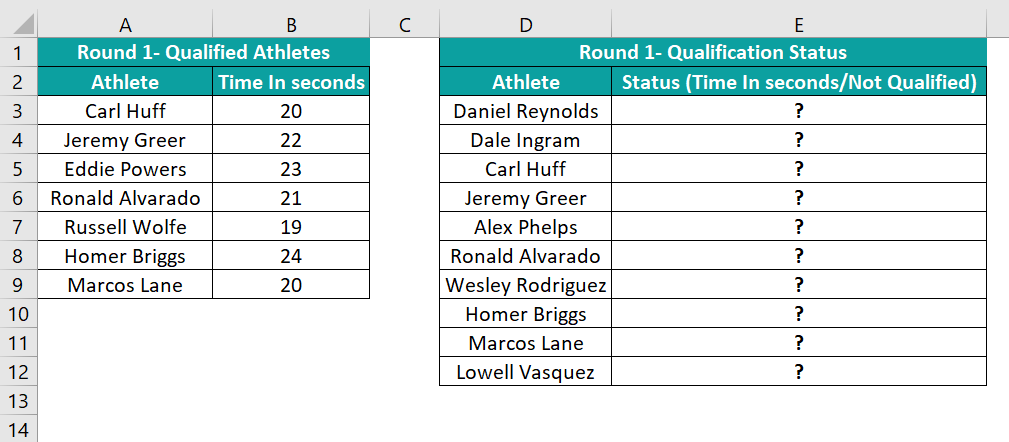Suppose we need to update the qualification status of all the athletes in column D and display the data in column E of the second table. Then, we can perform the calculations in the target cells using the ISERROR() with the functions MATCH, INDEX, and IF.

• Step 1: Select the target cell E3, enter the below formula, and press Enter.
=IF(ISERROR(INDEX(\$B\$3:\$B\$9,MATCH(D3,\$A\$3:\$A\$9,0))),”Not Qualified”,INDEX(\$B\$3:\$B\$9,MATCH(D3,\$A\$3:\$A\$9,0)))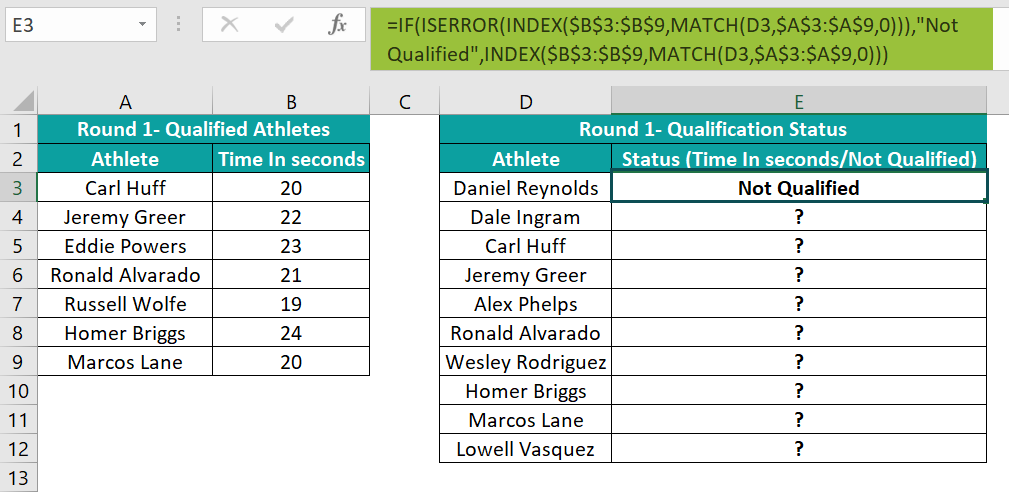• Step 2: Drag the fill handle downwards to copy the formula in cells E4:E12.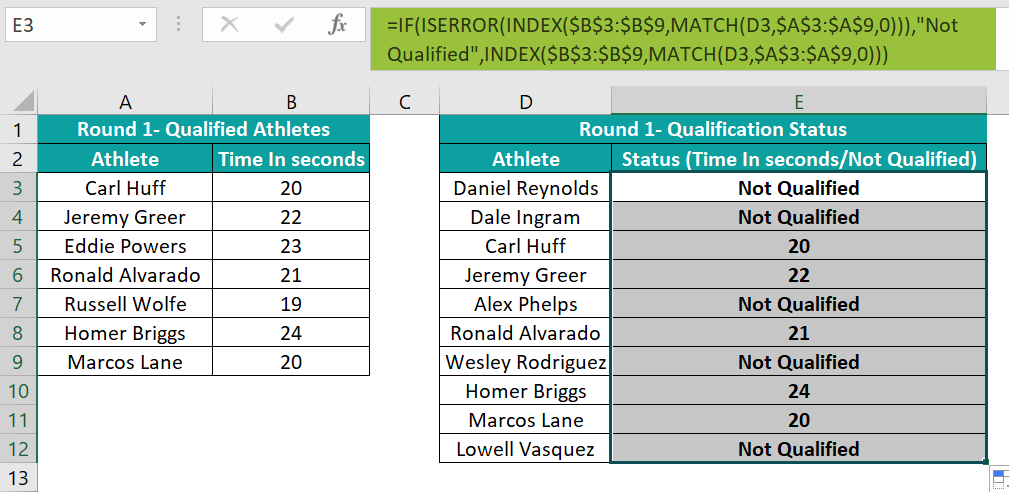Let us see how the formula works using the target cell E12 expression.

First, the MATCH() inside the INDEX(), within the ISERROR(), searches the name in cell D12, Lowell Vasquez, in the list of qualified athletes in column A. As there is no match, the function returns the #N/A error. And as the INDEX() has to check for the #N/A error in the cell range B3:B9, it also returns the #N/A error. Now, since the input to the ISERROR() is the #N/A error, it returns TRUE. Finally, the IF condition is true; thus, its output displayed in the target cell D12 is the term Not Qualified.

2. How do I use ISERROR in Excel VBA?

We can use ISERROR in Excel VBA using the method, IsError(expression).

We shall see the steps with an example.
Consider the following table. Column A contains a set of input data.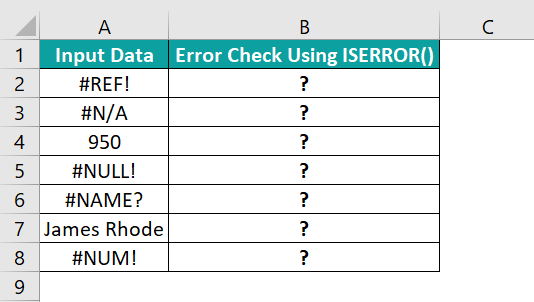And suppose we have to perform an error check for the given data and display the output in column B. Then, here is how we can apply the ISERROR() in Excel VBA and populate the specific target cells.

• Step 1: With the worksheet containing the above table open, press the keyboard shortcut Alt + F11 to open the VBA Editor.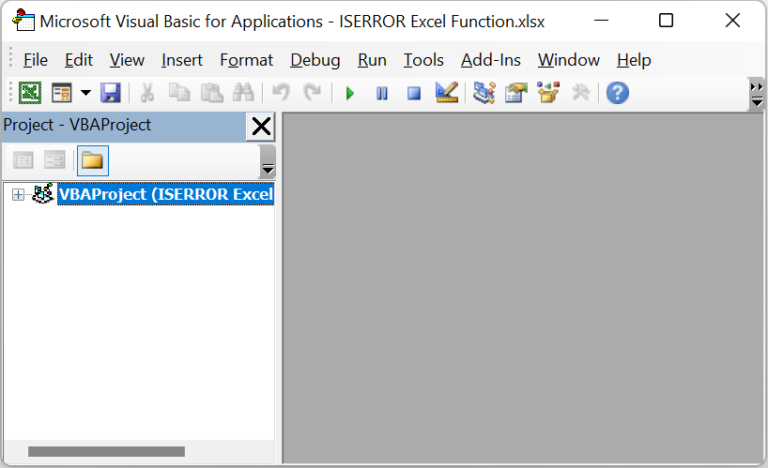• Step 2: Choose the required VBAProject from the left menu and select the option Module under the Insert tab to open the Module1 window.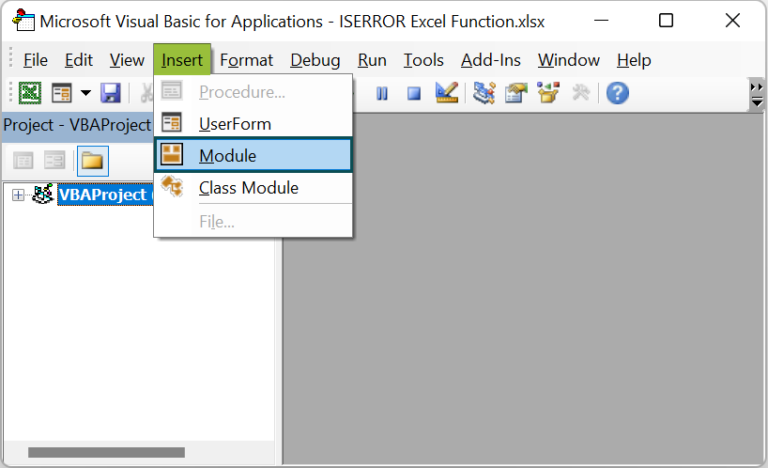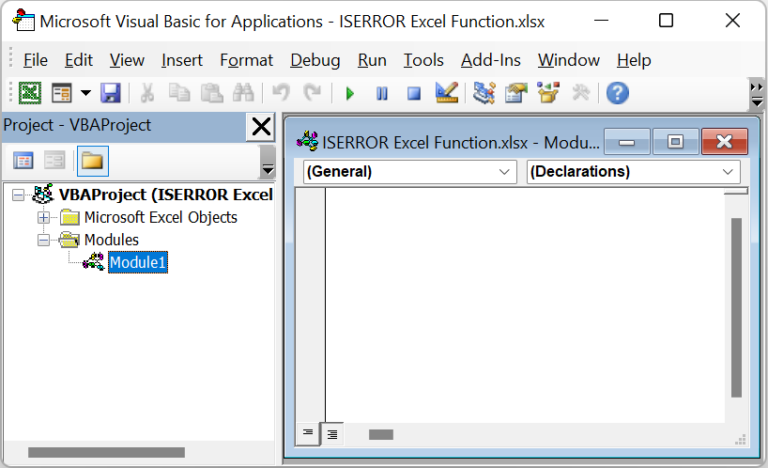• Step 3: Type the VBA code in the Module1 window to apply the ISERROR() in the specific target cells in the active worksheet.

Sub ISERROR_fn()

Range(“B2”) = IsError(Range(“A2”))
Range(“B3”) = IsError(Range(“A3”))
Range(“B4”) = IsError(Range(“A4”))
Range(“B5”) = IsError(Range(“A5”))
Range(“B6”) = IsError(Range(“A6”))
Range(“B7”) = IsError(Range(“A7”))
Range(“B8”) = IsError(Range(“A8”))

End Sub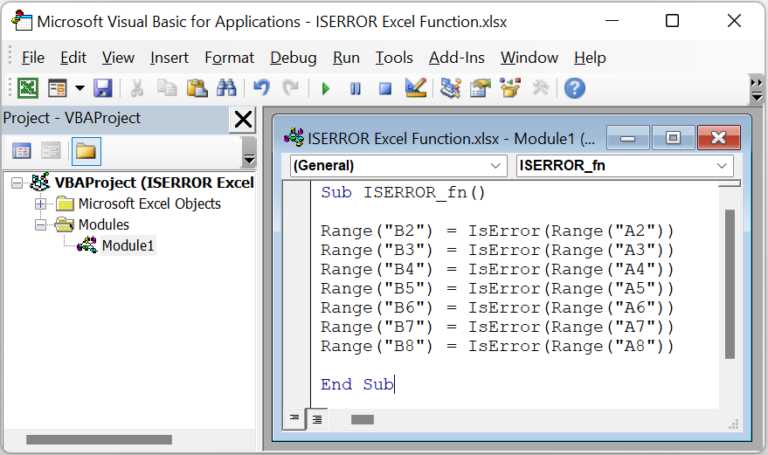• Step 4: Click the Run Sub/UserForm icon in the menu to execute the VBA code entered in the Module1 window.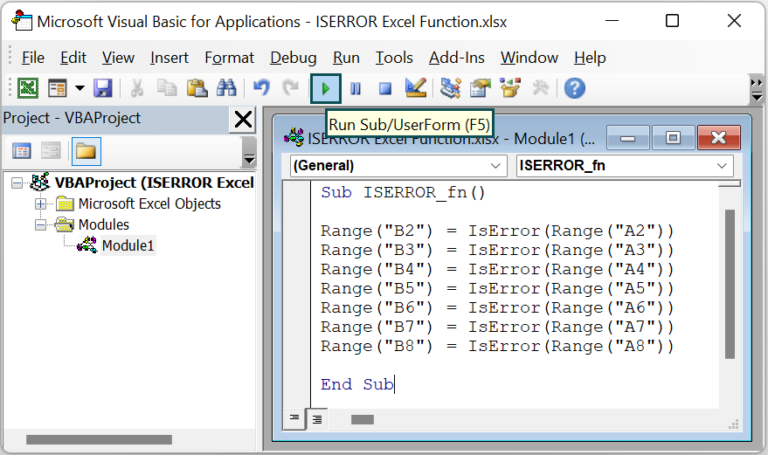We can now open the active worksheet to see the error check data populated in the target cells B2:B8.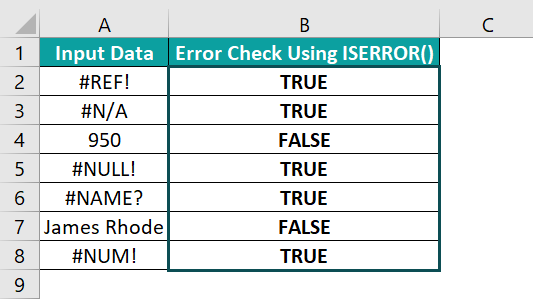The ISERROR() used in each command line accepts the cell address of the corresponding data in column A. And if the specified data is an error value, the function returns TRUE, which gets assigned to the respective target cell. On the other hand, the function returns FALSE when the given data in column A is not an error value.

3. What is the difference between the ISERROR() and IFERROR()?

The difference between ISERROR() and IFERROR() is that the ISERROR() checks if the given value is an error or not, returning a logical value accordingly. On the other hand, the IFERROR() returns the value you specify when the input test expression or data is an error and the outcome of the tested expression otherwise.